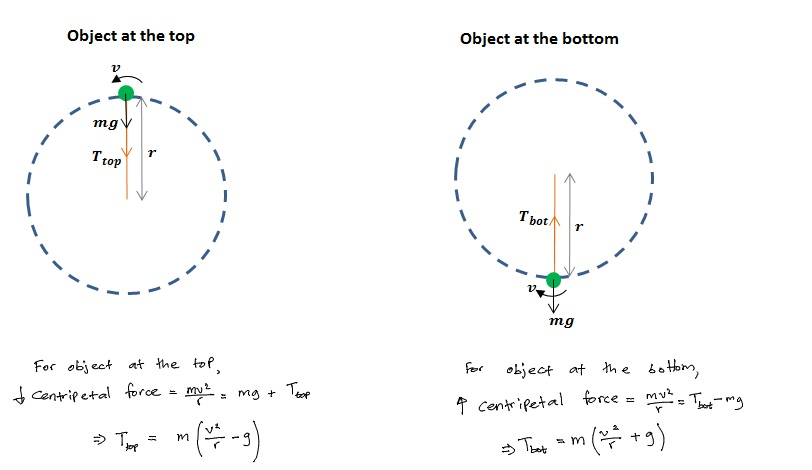# How to Solve Vertical Circular Motion Problems

In this article, we will look at how to solve vertical circular motion problems. The principles used to solve these problems are the same as those used to solve problems involving centripetal acceleration and centripetal force. Unlike with horizontal circles, the forces acting on vertical circles vary as they go around. We will consider two cases for objects moving in vertical circles: when objects move at constant speed and when they move at varying speeds.

## How to Solve Vertical Circular Motion Problems for Objects Traveling at a Constant Speed

If an object is traveling at a constant speed in a vertical circle, then the centripetal force on the object,$\frac{mv^2}{r}$ remains the same. For example, let’s think of an object with mass$m$ that is swung about in a vertical circle by attaching it to a string of length$r$. Here, then,$r$ is also the radius for the circular motion. There will be a tension$T$ always acting along the string, pointed towards the center of the circle. But the value of this tension will constantly vary, as we will see below.Vertical Circular Motion of an Object at Constant Speed v

Let us consider the object when it is at the top and the bottom of its circular path. Both the object’s weight,$mg$, and the centripetal force (pointed at the centre of the circle) remain the same.How to Solve Vertical Circular Motion Problems – Constant Speed Object Tension at Top and Bottom

The tension is greatest when the object is at the bottom. This is where the string is most likely to break.

## How to Solve Vertical Circular Motion Problems for Objects Traveling at a Varying Speed

For these cases, we consider the change in energy of the object as it travels around the circle. At the top, the object has most potential energy. As the object comes down, it loses potential energy, which is converted into kinetic energy. This means that the object speeds up as it comes down.

Suppose an object attached to a string moves in a vertical circle with varying speed such that, at the top the object has just enough speed$v_{top}$ to maintain its circular path. Below, we will derive expressions for this object’s minimum speed at the top, the maximum speed (when it is at the bottom) and the tension of the string when it is at the bottom.

At the top, the centripetal force is downward and$\frac{m{v_{top}}^2}{r}=T_{top}+mg$. The object will have just enough speed to maintain its circular path if the string is just about to go slack when it is at the top. For this case, the tension of the string$T_{top}$ is almost 0. Inserting this into the centripetal force equation, we will have$\frac{m{v_{top,\:min}}^2}{r}=mg$. Then,$v_{top,\:min}=\sqrt{gr}$.

When the object is at the bottom, its kinetic energy is greater. The gain in kinetic energy is equal to the loss in potential energy. The object falls through a height of$2r$ when it reaches the bottom, so the gain in kinetic energy is$2mgr$. Then,$\frac{1}{2}m{v_{bot}}^2=\frac{1}{2}m{v_{top}}^2+2mgr$.

Since our$v_{top}=\sqrt{gr}$, we have$\frac{1}{2}m{v_{bot}}^2=\frac{1}{2}m{\left({\sqrt{gr}}\right)}^2+2mgr$$\Rightarrow\frac{1}{2}m{v_{bot}}^2=\frac{1}{2}mgr+2mgr$$\therefore v_{bot}=\sqrt{5gr}$

Next, we look at the tension of the string at the bottom. Here, the centripetal force is directed upward. We then have$T_{bot}-mg=\frac{m{v_{bot}}^2}{r}$. Substituting$v_{bot}=\sqrt{5gr}$, we get$T_{bot}=mg+\frac{mgr}{r}$.

Simplifying further, we end up with:$T_{bot}=6mg$.

## Vertical Circular Motion Problems – Example

### Swinging Buckets of Water Overhead

A bucket of water can be swung overhead without the water falling down if it is moved at a large enough speed. The weight$mg$ of the water is trying to pull the water down; however, the centripetal force$\frac{mv^2}{r}$ is trying to keep the object in the circular path. The centripetal force itself is composed of the weight plus the normal reaction force acting on the water. Water will stay on the circular path as long as$\frac{mv^2}{r}>mg$.How to Solve Vertical Circular Motion Problems – Swinging a Bucket of Water

If the speed is low, such that$\frac{mv^2}{r}, then not all of the weight is “used up” to create the centripetal force. The downwards acceleration is greater than the centripetal acceleration, and so the water will fall down.

The same principle is used to maintain objects from falling when they go through “loop the loop” motions as seen in, for example, roller-coaster rides and in airshows where stunt pilots fly their aeroplanes in vertical circles, with the aeroplanes traveling “upside down” when they reach the top.

Example 1

The London Eye is one of the largest Ferris wheels on Earth. It has a diameter of 120 m, and rotates at a rate of about 1 complete rotation per 30 minutes. Given that it moves at a constant speed, Find

a) the centripetal force on a passenger of mass 65 kg

b) the reaction force from the seat when the passenger is at the top of the circle

c) the reaction force from the seat when the passenger is at the bottom of the circle

Note: In this particular example, the reaction force changes by very little, because the angular speed is quite slow. However, note that the expressions used to calculate the reaction forces at the top and bottom are different. This means the reaction forces would be considerably different when larger angular speeds are involved. The largest reaction force would be felt at the bottom of the circle.Vertical Circular Motion Problems – Example – The London Eye

Example 2

A bag of flour with a mass of 0.80 kg is swung about in a vertical circle by a string 0.70 m long. The speed of the bag varies as it travels around the circle.

a) Show that a minimum speed of 3.2 m s-1 is sufficient to maintain the bag in the circular orbit.

b) Calculate the tension in the string when the bag is at the top of the circle.

c) Find the speed of the  bag at an instant when the string has moved downwards by an angle of 65o from the top.How to Solve Vertical Circular Motion Problems – Example 2